• 01. 目的 求一元二次方程 ax2+bx+c=0ax2+bx+c=0ax^2+bx+c=0 的根，分...由代数基本定理，一元二次方程有且仅有两个根（重根按重数计算）：x=−b±b2−4ac−−−−−−−√2ax=−b±b2−4ac2a x = \dfrac{-b \pm \...
01. 目的
求一元二次方程 $ax^2+bx+c=0$ 的根，分情况讨论，结果保留2位小数。
02.  一元二次方程$ax^2+bx+c=0$ 的根
由代数基本定理，一元二次方程有且仅有两个根（重根按重数计算）：$x = \dfrac{-b \pm \sqrt{b^2 - 4ac}}{2a}$根的情况由判别式$\sqrt{b^2 - 4ac}$决定 ,利用一元二次方程根的判别式$\sqrt{b^2 - 4ac}$可以判断方程的根的情况。
一元二次方程  的根与根的判别式 有如下关系：
①当$\sqrt{b^2 - 4ac}> 0$   时，方程有两个不相等的实数根；
②当  $\sqrt{b^2 - 4ac}=0$时，方程有两个相等的实数根；
③当  $\sqrt{b^2 - 4ac}< 0$ 时，方程无实数根，但有2个共轭复根。
上述结论反过来也成立。
方程的形式与系数a、b、c的关系
① 如果系数a、b、c都为0，则输出“方程不存在！"；
② 如果a和b为0，c不为0，此时c=0不是方程，输出"方程不存在！"；
③  如果当a为0，且b、c不为0时,方程变为一元一次方程bx+c=0,此时方程
只有一个解；
④根据判别式确定方程的解。
###03. 求根代码
package com.test;//创建一个test包

import java.util.Scanner;//导入java.util包中的Scanner类(使用此类可以方便的完成输入流的输入操作)
import java.math.BigDecimal;//导入java.math包中的BigDecimal(用来对超过	16位有效位的数进行精确的运算)

//对一元二次方程中各系数的不同情况作讨论，列出不同情况下根的分布，最终求出存在的根
public class Squ { //定义一个公共类---Squ
public static void main(String[]args)
{
double X1,X2;//定义临时变量X1，X2;
System.out.println("方程表达式为：ax2＋bx＋c＝0");//显示台输出---方程表达式为：ax2＋bx＋c＝0
@SuppressWarnings("resource")
Scanner sc=new Scanner(System.in);//用Scanner类获取控制台的输入(即获得从键盘输入的数)
System.out.println("请输入a的值：");//显示台输出提示---输入a的值
//每一次从键盘输入值后回车执行后面的内容
double a=sc.nextDouble();//接受控制台输入的数字给形参a赋值
System.out.println("请输入b的值：");//显示台输出提示---输入b的值
double b=sc.nextDouble();//接受控制台输入的数字给形参b赋值
System.out.println("请输入c的值：");//显示台输出提示---输入c的值
double c=sc.nextDouble();//接受控制台输入的数字给形参c赋值
double t=b*b-4*a*c;//定义一个变量t,且t=b*b-4*a*c,此时t为方程根的判别式

//用判断语句对方程的系数的不同情况作讨论，并得出根的分布情况
//① 判断当a、b、c都为0时，方程变为等式0=0;方程不存在！
if(a==0 && b==0 && c==0)
{
System.out.println("方程不存在！");//显示台输出
}
//② 当a、b都为0，而c不为0时，方程变为等式c=0,此中不含未知数，方程不存在！
else if(a==0 && b==0 && c!=0)
{
System.out.println("方程不存在！");//显示台输出
}
//③ 当a为0，且b、c不为0时,方程变为一元一次方程bx+c=0,此时方程只有一个解
else if(a==0 && b!=0 && c!=0)
{
System.out.println("此方程为一元一次方程");//显示台输出
double result = (-1 * c) / b;//定义一个变量result存放方程的解;此时解为：-c/b
System.out.println(resetValue(result)+"方程的解为：");
}
//④ 以上定义了t=b*b-4*a*c，若t>0,则t的开方为实数，此时方程有两个不同的实根，且两实根为相反数
else if(t>0)
{
System.out.println("方程有两个实根");//显示台输出
//第一根的算法：((-b) + Math.sqrt(t)) / 2 * a( Math.sqrt是指math方法中的sqrt类，即开方)
X1= ((-b) + Math.sqrt(t)) / 2 * a;
//第一根的算法：((-b) - Math.sqrt(t)) / 2 * a
X2= ((-b) - Math.sqrt(t)) / 2 * a;
//显示台输出两根的值
System.out.println("X1="+resetValue(X1));
System.out.println("X2="+resetValue(X2));
}
//⑤ 如果t<0,则t开方后产生虚数，此时方程有一对共轭副根
else if(t<0)
{
double X4,X5;//定义临时变量X4、X5
//⑥ 如果b不等于0，则其根为共轭副根，根由虚部和实部组成
if(b!=0)
{
X4=(-1*b)/(2*a);//根的实部
X5=Math.sqrt(-1*t)/(2*a);//根的虚部
System.out.println("方程有一对共轭副根：");//显示台输出
//输出时toString()表示用科学计数法输出根的值;加i指虚部的表示法;其中的"+、-"连接根的实部和虚部，形成共轭
System.out.println("X1="+resetValue(X4).toString()+"+"
+resetValue(X5).toString()+"i");//输出第一个根的值
System.out.println("X2="+resetValue(X4).toString() + "-"
+ resetValue(X5).toString() + "i");//输出第二个根的值
}
//⑦ 若b=0,则此时根只有虚部没有实部，是一对共轭副根，实部为0
else
{
double X6=Math.sqrt(-1*t)/(2*a);//根中只有虚部
System.out.println("方程有一对相反虚根：");//显示台输出
//输出时toString()表示用科学计数法输出根的值;加i指虚部的表示法;其中的"+、-"连接根的实部和虚部，形成共轭，此时实部为0
System.out.println("X1=0-"+resetValue(X6).toString()+"i");
System.out.println("X2=0+"+resetValue(X6).toString()+"i");

}
}
//⑧ 若t=0,则方程有一个实根
else if(t==0)
{
double X3=(-1*b)/2*a;//根的计算
System.out.println("方程有一个实根");//显示台输出
System.out.println("方程的解为："+resetValue(X3));//显示台输出方程的解
}

}

//使用静态方法将定义的值由double类型转换为BigDecimal类型(声明)
private static BigDecimal resetValue(double n) {
// TODO 自动生成的方法存根
BigDecimal bd=new BigDecimal(n);//将n的值赋给形参bd
//返回的值保留两位小数，默认用四舍五入方式 （ROUND_HALF_UP表示遇5进1；setScale规定要保留的小数位数）
return bd.setScale(2,BigDecimal.ROUND_HALF_UP);
}

}


###04. 运行结果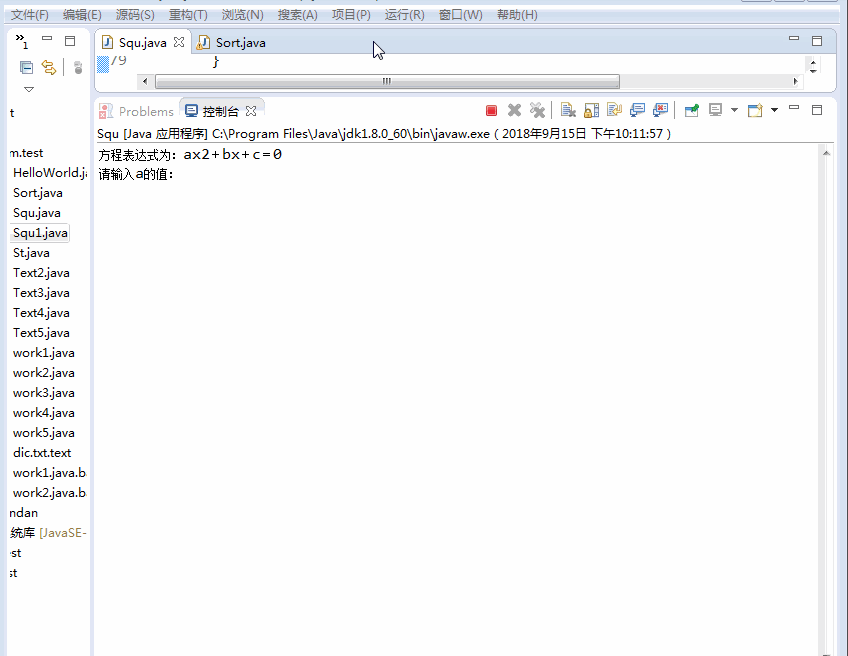展开全文java
• * @desc 一元三次方程，二次方程，和一次方程求解工具类 */ public class EquationCalculation { /* 计算一元三次方程最大实 * 一元三次方程(ax3+bx2+cx+d＝0)的盛金公式解题法 * 输入：参数a,b,c,d * 1)：...
/**
* @author Along
* @desc 一元三次方程，二次方程，和一次方程求解工具类
*/
public class EquationCalculation {

/* 计算一元三次方程最大实根
* 一元三次方程(ax3+bx2+cx+d＝0)的盛金公式解题法
* 输入：参数a,b,c,d
* 1)：当A=B=0时，方程有一个三重实根；
* 2)：当Δ=B^2－4AC>0时，方程有一个实根和一对共轭虚根,只处理实根；
* 3)：当Δ=B^2－4AC=0时，方程有三个实根，其中有一个两重根；
* 4)：当Δ=B^2－4AC<0时，方程有三个不相等的实根。
* 返回 : 所有根中大于0的最大值，不存在大于0的值时方程无有效解或有误，返回异常
*/
public static double solveCubic(double a, double b, double c, double d)  throws ArithmeticException{
double t1=0,t2=0,t3=0;
if (a != 0) {
double A = b * b - 3 * a * c; // A=b*b-3ac
double B = b * c - 9 * a * d; // B=bc-9ad
double C = c * c - 3 * b * d; // C=c*c-3bd
double D = B * B - 4 * A * C; // 判别式D=B*B-4*A*C
if (A == 0 && B == 0) {
//当A=B=0时，盛金公式1： t1=t2=t3=－b/(3a)=－c/b=－3d/c
t1 = -c / b;
t2 = t1;
t3 = t1;
}else{
if (D > 0) {
/*
* 当D=B^2－4AC>0时，盛金公式2：t1=(－b－(Y1)^(1/3)－(Y2)^(1/3))/(3a)；
* t2，t3=(－2b＋(Y1)^(1/3)＋(Y2)^(1/3))/(6a)±i3^(1/2)((Y1)^(1/3)－(Y2)^(1/3))/(6a)，
* 其中Y1，Y2=Ab＋3a(－B±(B^2－4AC)^(1/2))/2，
* 因i^2=－1，故t2和t3为虚根，忽略不使用
*/
double Y1 = A * b + 3 * a * (-B + Math.sqrt(D)) / 2;
double Y2 = A * b + 3 * a * (-B - Math.sqrt(D)) / 2;
// Math.pow(a,b)使用时a不能为负数，分情况判断计算
if(Y1<0) {
Y1=-Math.pow(Math.abs(Y1), 1.0 / 3);
}else {
Y1=Math.pow(Math.abs(Y1), 1.0 / 3);
}
if(Y2<0) {
Y2=-Math.pow(Math.abs(Y2), 1.0 / 3);
}else {
Y2=Math.pow(Math.abs(Y2), 1.0 / 3);
}

double F =Y1 +Y2;
t1 = (-b - F) / (3 * a);
t2 = t1;
t3 = t1;
}else if (D == 0) {
/*
* 当D=B^2－4AC=0时，盛金公式3：t1=－b/a＋K； t2=t3=－K/2，
* 其中K=B/A，(A≠0)。
* 盛金定理：当D=0时，若B≠0，盛金公式3一定不存在A≤0的值，故不需要判断A是否等于0
* */
double K = B / A;
t1 = -b / a + K;
t2 = -K / 2;
t3 = t2;
}else{
/*
* 当D=B^2－4AC<0时，盛金公式4： t1=(－b－2A^(1/2)cos(θ/3))/(3a)；
* t2，t3=(－b＋A^(1/2)(cos(θ/3)±3^(1/2)sin(θ/3)))/(3a)，
* 其中θ=arccosT，T=(2Ab－3aB)/(2A^(3/2))，(A>0，－1<T<1)。
* 盛金定理：当D<0时，盛金公式4一定不存在A<=0的值，且一定不存在T<=-1或T>=1的值，故条件A>0和－1<T<1不需要判断
* */
double T = (2 * A * b - 3 * a * B) / (2 * Math.sqrt(A * A * A));
double q = Math.acos(T);
double q3 = q / 3.0;
t1 = (-b - 2 * Math.sqrt(A) * Math.cos(q3)) / (3 * a);
t2 = (-b + Math.sqrt(A) * Math.cos(q3) - Math.sqrt(A) * Math.sqrt(3) * Math.sin(q3)) / (3 * a);
t3 = (-b + Math.sqrt(A) * Math.cos(q3) + Math.sqrt(A) * Math.sqrt(3) * Math.sin(q3)) / (3 * a);
}
}
//返回多个结果中大于0的最大值，若都小于0，则返回0
double jg[]={t1,t2,t3};
Arrays.sort(jg);
if(jg < 0){
//方程无大于等于0的有效实根，返回0
throw new ArithmeticException("方程无大于等于0的有效实根!");
}else{
return jg;
}
}else{
//a=0时,为一元二次方程，调用一元二次方程求根函数
return solveQuadratic(b,c,d);
}
}

/* 计算一元二次方程最大实根
* 一元三次方程(ax2+bx+c＝0)的公式解题法
* 输入：参数a,b,c
* 1)：当Δ=b^2－4ac<0时，方程有无实根；
* 2)：当Δ=b^2－4ac=0时，方程有两个相同的根为-b/2a；
* 3)：当Δ=b^2－4ac<0时，方程有两个根为(-b±(b^2-4ac)^(1/2))/(2a)。
* 返回 : 所有根中大于0的最大值根，不存在大于0的值时方程无有效解或有误，返回异常
*/
public static double solveQuadratic(double a,double b,double c) throws ArithmeticException{
double t1=0,t2=0;
if(a!=0){
double A = b * b - 4 * a *c;
if(A < 0){
//方程无实根，返回0
throw new ArithmeticException("方程无实根!");
}else if(A == 0){
t1=t2= -b/(2*a);
}else{
t1= (-b + Math.pow(A, 1.0/2)) / (2 * a);
t2= (-b - Math.pow(A, 1.0/2)) / (2 * a);
}
//返回多个结果中大于0的最大值，若都小于0，则返回0
double jg[]={t1,t2};
Arrays.sort(jg);
if(jg <= 0){
//方程无大于等于0的有效实根，返回0
throw new ArithmeticException("方程无大于等于0的有效实根!");
}else{
return jg;
}
}else{
//一元一次方程
return solveLinear(b,c);

}
}

/* 计算一元一次方程实根
* 一元一次方程(ax+b＝0)的公式解题法
* 输入：参数a,b
* 当a！=0，且b！=0时，x=-b/a;
* 当b=0时，x=0;
* 返回 : 大于0的实根，不存在大于0的值时方程无有效解或有误，返回异常
*/
public static double solveLinear(double a,double b) throws ArithmeticException{
double t1=0;

if(b == 0){
//方程等式无意义，返回0
throw new ArithmeticException("方程等式无意义!");
}else {
if(a!=0){
t1=-b/a;
if(t1 < 0){
//方程无大于等于0的有效实根，返回0
throw new ArithmeticException("方程无大于等于0的有效实根!");
}else{
return t1;
}
}else{
//a=0且b！=0,方程等式不成立，返回异常信息
throw new ArithmeticException("方程等式不成立!");
}
}
}
}


展开全文java 算法
• 简单C语言小程序：求根公式求一元二次方程式的根！...有两个重根（大小相等的根）或者两个大小不等的根，为了是程序更加完善还要考虑到a =0的情况，即此时不能看做一元二次方程而只能将其看作一...
简单C语言小程序：求根公式求一元二次方程式的根！
一、算法的构思
一元二次方程的一般式：ax^2+bx+c = 0
我们知道，一元二次方程有解（根）的充要条件是：b^2-4ac>=0。如果不满足此关系式，那么方程无解。接着当方程有解的时候又出现了两种情况：1.有两个重根（大小相等的根）或者两个大小不等的根，为了是程序更加完善还要考虑到a =0的情况，即此时不能看做一元二次方程而只能将其看作一元一次方程，本程序运用求根公式来实现功能，有兴趣的伙计可以试试下面给出的韦达定理实现功能。
求根公式：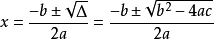扩展——韦达定理：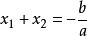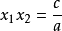二、全部代码
#include <stdio.h>
#include <math.h>
double a = 0, b = 0, c = 0, p, q;
int main(void)
{
printf("请分别输入a,b,c三个值并用空格隔开，按回车开始运算：\n");
scanf("%lf %lf %lf", &a, &b, &c);
if(a == 0 )
printf("此一元一次方程的解为x= %lf\n", -c/b);
else if (b*b-4*a*c<0)
printf("此方程无解\n");
else
{
p = -b/2*a;
q = (sqrt(b*b-4*a*c)/2*a);
printf("此一元二次方程的解为x1= %lf,x2= %lf\n该抛物线的对称轴为：= %lf\n最大/最小值为: = %lf", p+q,p-q, -b/2*a,(4*a*c-b*b)/4*a);
}
system("pause");
return 0;
}


三、给生成的程序添加一个漂亮的图标
我们知道，当C编译完成之后就会有一个默认的图标，很难看，如图：
那么我们如何可以给它换一个漂亮的图标呢？
1.首先，要去网上搜集几个你中意的ico图片文件来当他的图标。
2.在DEV C++左侧项目管理功能栏中找到项目，单击右键，弹出一个选择框，选择项目属性。
这时我们可以看到左下角有图标选项，点击浏览把从网上找来的ico文件选择进去再进行编译一次就可以了。
最后谢谢浏览，欢迎指正！


展开全文算法 c语言
• 我这里主要是用著名的盛金公式解一元三次方程，不得不说盛金公式更为直观性，与我们解一元二次方程很类似。下面就拿来直接用了。 盛金公式 重根判别式：，总判别式为：。还有四个求根公式详见给的链接，这里...

引言

一元三次方程的解法有多种，百度百科也有介绍，卡尔丹公式和盛金公式。我这里主要是用著名的盛金公式解一元三次方程，不得不说盛金公式更为直观性，与我们解一元二次方程很类似。下面就拿来直接用了。

盛金公式

重根判别式：，总判别式为：。还有四个求根公式详见给的链接，这里就不一一写出了。

代码实现

C++

从上可以看出，转成C++/matlab很容易实现，但是注意C++判断一个数（float）等于0不能用==0，而是用<1e-6，所有有时候会有一些很小的误差。下面我给出我的实现函数ShengJin，调用即可。注意这里我只取出了实根。

void ShengJin(double a,double b,double c,double d,vector<double> &X123)
{
/************************************************************************/
/* 盛金公式求解三次方程的解
德尔塔f=B^2-4AC
这里只要了实根，虚根需要自己再整理下拿出来
*/
/************************************************************************/
double A=b*b-3*a*c;
double B=b*c-9*a*d;
double C=c*c-3*b*d;
double f=B*B-4*A*C;
double i_value;
double Y1,Y2;
if (fabs(A)<1e-6 && fabs(B)<1e-6)//公式1
{
X123.push_back(-b/(3*a));
X123.push_back(-b/(3*a));
X123.push_back(-b/(3*a));
}
else if (fabs(f)<1e-6)   //公式3
{
double K=B/A;
X123.push_back(-b/a+K);
X123.push_back(-K/2);
X123.push_back(-K/2);
}
else if (f>1e-6)      //公式2
{
Y1=A*b+3*a*(-B+sqrt(f))/2;
Y2=A*b+3*a*(-B-sqrt(f))/2;
double Y1_value=(Y1/fabs(Y1))*pow((double)fabs(Y1),1.0/3);
double Y2_value=(Y2/fabs(Y2))*pow((double)fabs(Y2),1.0/3);
X123.push_back((-b-Y1_value-Y2_value)/(3*a));//虚根我不要
//虚根还是看看吧，如果虚根的i小于0.1，则判定为方程的一根吧。。。
i_value=sqrt(3.0)/2*(Y1_value-Y2_value)/(3*a);
if (fabs(i_value)<1e-1)
{
X123.push_back((-b+0.5*(Y1_value+Y2_value))/(3*a));
}
}
else if (f<-1e-6)   //公式4
{
double T=(2*A*b-3*a*B)/(2*A*sqrt(A));
double S=acos(T);
X123.push_back((-b-2*sqrt(A)*cos(S/3))/(3*a));
X123.push_back((-b+sqrt(A)*(cos(S/3)+sqrt(3.0)*sin(S/3)))/(3*a));
X123.push_back((-b+sqrt(A)*(cos(S/3)-sqrt(3.0)*sin(S/3)))/(3*a));
}
}

这里参照博客matlab实现一元三次方程来验证代码。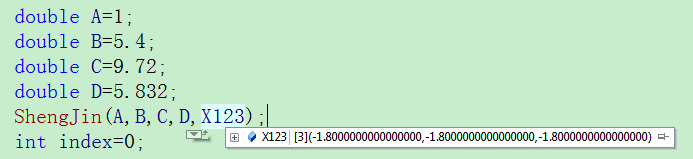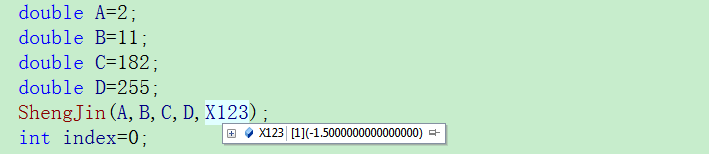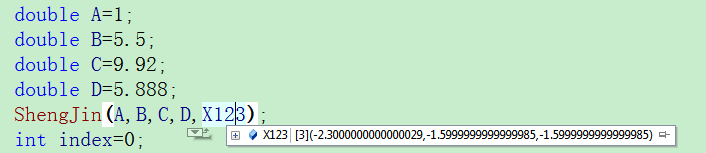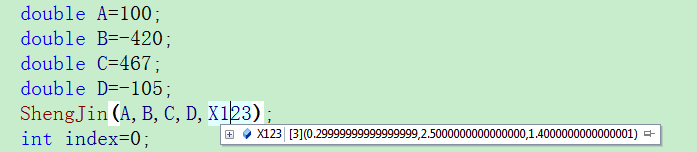matlab

matlab有自带的函数多项式求解函数：roots 求解一元三次方程。

x=roots([a, b, c, d]);
for k=1:length(x)
xx(k) = isreal(x(k));%求实根
end
X_real=x(xx); %X_real都是实根


当然，matlab也有大佬实现了盛金公式求解一元三次方程，可以参考这篇博客，写的很详细：盛金公式matlab版。

总结

在编写求解代码时，也看过和实践过别人的求解代码，很多都是用基于一次二次导数的二分法来求解方程（我还有一份，需要的可以私信我），较为复杂且费时。因此参照盛金公式进行了编写C++代码，网上肯定也有同类代码，思想都一样的，很多时候我们为了求解一元三次方程并不是实验，而是为了达到我们的研究目的，比如我这只要实根。

展开全文• 高中方程主要是熟练掌握一元二次方程，包括是否有实数解，是否重根等。三次方程求解只涉及较浅的部分。三次方程也有韦达定理和求根公式，但是不要求掌握。对于高考中出现的三次方程求解，不要慌张，按部就班的通过试...数学建模
• 从题目应该可以看出是，一个一元二次方程求解的问题，但如何解呢？ 首先明白什么是实根与重根？ 单根是指特征方程只有一个单实根,即只有一个实数解. 重根是指特征方程的解中有相等的根,那么相等的根就称为方程的一...
• 1. 输入一元二次方程ax2＋bx＋c＝0的系统a、b、c，计算输出一元二次方程的两个根x1，x2。界面如下图所示。提示：求根时需要考虑重根、复根的情况；以及在用Math.Sqrt（ ）函数求平方根时需要考虑避免是负数的情况。计算
• 一元二次方程ax2+bx+c=0的，三个系数a, b, c由键盘输入，且a不能为0，但不保证b2-4ac&gt;0。 程序中所涉及的变量均为double类型。 输入 以空格分隔的一元二次方程的三个系数，双精度double类型 输出 ...
• 任何复系数一元n多项式 方程在复数域上至少有一根(n≥1)，由此推出，n复系数多项式方程在复数域内有且只有n个根（重根按重数计算） p(x)=an(x−z1)(x−z2)(x−z3)…(x−z1),zi∈C {\color{Purple}p (x) = a _{n}...
• 1．求一元二次方程的根。 要求：输入一元二次方程的三个系数a、b、c，根据系数值，可得出如下三种根: (1)△>0，两个实根 (2)△=0，重根,即相同根 (3)△<0，无实数根 输出两个实根x1和x2，若没有实根则输出信息...python 算法 数据结构
• 盛金公式 昨天做一道二分的题目，就是一元三次方程求三个根，然后从小到大输出。 我发现好多大佬都是用的盛金公式或者牛顿迭代(听说比二分快)。...首先和一元二次方程类似，他也有重根判别式：A=b*b-3*a*c; B...python 算法 机器学习 http
• 牛顿迭代法是求方程根的重要方法之一，其最大优点是在方程f(x) = 0的单根附近具有平方收敛，而且该法还可以用来求方程重根、复根。 牛顿法是方程求根的一个有力方法，常常能快速求出其他方法求不出或者难以求出...
• 教学难点: 算法的设计及意义 对于一元二次方程,可以用熟悉的求公式来求解,但是,绝大部分的方程不存在求公式. 在实际问题中,通常只要获得满足一定精确度的近似解就可以了.因此,讨论方程近似解的算法具有重要的...
• 10．4．1 二次规划函数quadprog 10．4．2 二次规划问题的应用 10．5 有约束最小化 10．5．1 有约束最小化函数fmincon 10．5．2 有约束最小化应用 10．6 目标规划 10．6．1 目标规划函数fgoalattain 10．6．2 目标...Matlab
• 非线性方程方程组的求解195 4.1 求非线性方程的对分法195 4.2 求非线性方程一个实的牛顿法198 4.3 求非线性方程一个实的埃特金迭代法201 4.4 求非线性方程一个实的试位法204 4.5 求非线性方程一个实的...
• ## C++常用算法程序集

热门讨论 2011-03-24 09:15:32
5.7 第种边界条件的三样条函数插值、微商与积分301 5.8 第三种边界条件的三样条函数插值、微商与积分307 5.9 二元Lagrange插值314 5.10 最小二乘曲线拟合319 5.11 切比雪夫曲线拟合326 5.12 最佳一致逼近的里...
• 第8章 插值与逼近 8.1 一元全区间插值 8.2 一元三点插值 8.3 连分式插值 8.4 埃尔米特插值 8.5 特金逐步插值 8.6 光滑插值 8.7 第一种边界条件的三样条函数插值 8.8 第种边界条件的三样条函数插值 8.9 第三种...
• 7.3 求非线性方程一个实的埃特金矢代法 7.4 求非线性方程一个实的连分法 7.5 求实系数代数方程全部的QR方法 7.6 求实系数方程全部的牛顿下山法 7.7 求复系数方程的全部牛顿下山法 7.8 求非线性方程组一组实...
• 7.3 求非线性方程一个实的埃特金矢代法 7.4 求非线性方程一个实的连分法 7.5 求实系数代数方程全部的QR方法 7.6 求实系数方程全部的牛顿下山法 7.7 求复系数方程的全部牛顿下山法 7.8 求非线性方程组一组实...
• 5.7 第种边界条件的三样条函数插值、微商与积分301 5.8 第三种边界条件的三样条函数插值、微商与积分307 5.9 二元Lagrange插值314 5.10 最小二乘曲线拟合319 5.11 切比雪夫曲线拟合326 5.12 最佳一致逼近的里...
• 7.3 求非线性方程一个实的埃特金矢代法 7.4 求非线性方程一个实的连分法 7.5 求实系数代数方程全部的QR方法 7.6 求实系数方程全部的牛顿下山法 7.7 求复系数方程的全部牛顿下山法 7.8 求非线性方程组一组实...
• 7.3 求非线性方程一个实的埃特金矢代法 7.4 求非线性方程一个实的连分法 7.5 求实系数代数方程全部的QR方法 7.6 求实系数方程全部的牛顿下山法 7.7 求复系数方程的全部牛顿下山法 7.8 求非线性方程组一组实...
• 7.3 求非线性方程一个实的埃特金矢代法 7.4 求非线性方程一个实的连分法 7.5 求实系数代数方程全部的QR方法 7.6 求实系数方程全部的牛顿下山法 7.7 求复系数方程的全部牛顿下山法 7.8 求非线性方程组一组实...
• 7.3 求非线性方程一个实的埃特金矢代法 7.4 求非线性方程一个实的连分法 7.5 求实系数代数方程全部的QR方法 7.6 求实系数方程全部的牛顿下山法 7.7 求复系数方程的全部牛顿下山法 7.8 求非线性方程组一组实...
• 7.2.2.齐次方程 7.2.3.一阶线性微分方程 7.2.4.伯努利方程 7.3.高阶方程 7.3.1.可降阶的高阶线性微分方程 7.3.2.高阶线性方程组 线性微分方程解的结论 常系数齐次线性微分方程的通解 常系数非齐次线性微分方程的通解...
• 非线性方程方程组的求解 7.1 求非线性方程一个实的对分法 7.2 求非线性方程一个实的牛顿法 7.3 求非线性方程一个实的埃特金矢代法 7.4 求非线性方程一个实的连分法 7.5 求实系数代数方程...
• 1.4.1 获得二叉树的深度和（顺序结构） 141 范例1-55 获得二叉树的深度和 141 ∷相关函数：BiTreeDepth函数 Root函数 1.4.2 获得二叉树的深度和（链表结构） 144 范例1-56 获得二叉树的深度和 144 ∷...
• 1.4.1 获得二叉树的深度和（顺序结构） 141 范例1-55 获得二叉树的深度和 141 ∷相关函数：BiTreeDepth函数 Root函数 1.4.2 获得二叉树的深度和（链表结构） 144 范例1-56 获得二叉树的深度和 144 ∷...
• 1.4.2 获得二叉树的深度和（链表结构） 144 范例1-56 获得二叉树的深度和 144 ∷相关函数：BiTreeDepth函数 Root函数 1.4.3 树的插入（顺序结构） 147 范例1-57 树的插入 147 ∷相关函数：InsertChild函数 ...